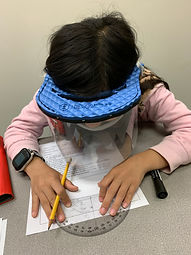## Ms. Kayla

### Target 1​

###### Lesson Type:

Review

Geometry

:

Shape

Draw geometric shapes with given conditions.

###### 1:

Using a protractor, create geometric shapes with given angles.

7th

###### Vocabulary:

Protractor, Degree, Geometric, Angle, Acute, Obtuse, Right angle, Parallel, Intersect, Conditions

Activities:

1. Students determined the degree of given angles the the use of a protractors.

2. Students created an image using a variety of conditions such as acute, obtuse, right angles and parallel and intersecting lines.

3. Students practiced labeling their angles.### Home Exploration

###### Challenge Problem:

How many degrees are there in your name?

###### Guiding Questions:

Students prints their name on a piece of paper and measures the angles they see.## Absent Students:

### Target 2

:

###### 1:

Represent quantities as ratios, fractions, decimals, or percentages.

###### 2:

Identify equivalent fractional parts (fractions, decimals, and percentages).

###### 3:

Convert given quantities into different fractional types, understanding that each manifestation is equivalent.

###### 4:

Convert fractions to their equivalent decimal form using division.

6th

###### Vocabulary:

Fraction, Decimal, Percentage, Equivalent, Convert, Remaining

Activities:

1. Students took turns "bowling" and identified the fraction, decimal, and percentage of the remaining pins.### Home Exploration

How many presents under the Christmas tree are yours? Represent it in a fraction, decimal, and percentage.

###### Guiding Questions:### Target 3

:

###### Vocabulary:

Activities:### Home Exploration